# RD Sharma Solutions - Chapter 22 - Mensuration - III (Part - 4), Class 8, Maths Notes | Study RD Sharma Solutions for Class 8 Mathematics - Class 8

## Class 8: RD Sharma Solutions - Chapter 22 - Mensuration - III (Part - 4), Class 8, Maths Notes | Study RD Sharma Solutions for Class 8 Mathematics - Class 8

The document RD Sharma Solutions - Chapter 22 - Mensuration - III (Part - 4), Class 8, Maths Notes | Study RD Sharma Solutions for Class 8 Mathematics - Class 8 is a part of the Class 8 Course RD Sharma Solutions for Class 8 Mathematics.
All you need of Class 8 at this link: Class 8

PAGE NO 22.27:

Question 37:

Find the cost of sinking a tubewell 280 m deep, having diameter 3 m at the rate of Rs 3.60 per cubic metre. Find also the cost of cementing its inner curved surface at Rs 2.50 per square meter.

Cost of sinking a tube well = Volume of the tube well  ×  Cost of sinking a tube well per cubic meter
=  22/7 x (1.52) x (280) m³ x Rs 3.6/m³  = Rs 7128.
Cost of cementing = Inner surface area of the tube well  ×  Cost of cementing per square meter
= ((2 x 22/7 x 1.5x 280) m2) x Rs 2.5/m2  = Rs 6600

Question 38:

Find the length of 13.2 kg of copper wire of diameter 4 mm, when 1 cubic cm of copper weighs 8.4 gm.

Since we know the weight and the volume of copper,  we can calculate its density.

density of copper =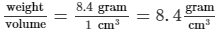If the weight of copper wire is 13.2 kg and the density of copper is 8.4 g/cm³, then:

Volume = Weight / Density = 13.2 kg x 1000 gram/kg / 8.4 gram/cm³  = 1571.43 cm³

The radius of copper wire is 2 mm or 0.2 cm.

So, the length of the wire can be determined in the following way: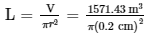= 125050.01 cm = 125 m

Thus, the length of 13.2 kg of copper is 125 m.

Question 39:

2.2 cubic dm of brass is to be drawn into a cylindrical wire 0.25 cm in diameter. Find the length of the wire.

Let r cm be the radius of the wire and h cm be the length of the wire.
Volume of brass = Volume of the wire
We know that the volume of brass = 2.2 dm³ = 2200 cm³
Volume of the wire =  πr²h  = (0.125 cm)2 (h)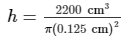= 44800 cm = 448 mThus, length of the wire is 448 m.

Question 40:

A well with 10 m inside diameter is dug 8.4 m deep. Earth taken out of it is spread all around it to a width of 7.5 m to form an embankment. Find the height of the embankment.

Let r m be the radius and d m be the depth of the well that is dug.

Volume of the well =  πr2d  = π(5 m)2(8.4 m) = 660 m³

An embankment has the shape of hollow cylinder with thickness. Its inner radii is equal to the well's radii,

i.e. r = 5 m, and its outer radii is R  = (5 + 7.5 ) = 12.5 cm.

Then, the volume of the embankment =  πh(R − r2)

Volume of the well = Volume of the embankment

659.73 m³  =  πh((12.5 m)2 − (5 m)2)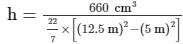= 1.6 m

Hence, the height of the embankment is 1.6 m.

Question 41:

A hollow garden roller, 63 cm wide with a girth of 440 cm, is made of 4 cm thick iron. Find the volume of the iron.

t  = Thickness = 4 cm
w  = Width = 63 cm

Girth = 440 cm =  2πR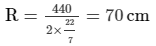r  =  R − t  =  70 cm − 4 cm = 66 cm
Volume of the iron =  π (R2 − r2) w  =  22/7 − (702 − 662) − (63) = 107712 cm³

Hence, volume of the iron is 107712 cm³.

Question 42:

What length of a solid cylinder 2 cm in diameter must be taken to recast into a hollow cylinder of length 16 cm, external diameter 20 cm and thickness 2.5 mm?

R  = External radius = 10 cm
h  = Length of the cylinder
t  = Thickness = 0.25 cm

Volume of the hollow cylinder =  πh(R2 - r2)

=  π (16) (102 - (10-0.25)2) = 79 π cm³

Volume of the solid cylinder = Volume of the hollow cylinder
We know that the radius of the solid cylinder is 1 cm.
∴ π(12)h  = 79 π
h  = 79 cm
Hence, length of the solid cylinder that gives the same volume as the hollow cylinder is 79 cm.

Question 43:

In the middle of a rectangular field measuring 30m × 20m, a well of 7 m diameter and 10 m depth is dug. The earth so removed is evenly spread over the remaining part of the field. Find the height through which the level of the field is raised.

Let r  m be the radius and h m be the depth of the well that is dug.
Volume of the well =  ππr2h  =  22/7  × (3.5 m)2  × (10 m) = 385 m³
Volume of the well = Volume of the rectangular field
Volume of the rectangular field =  385 m³  = 30 m × 20 m × height
Height =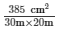= 0.641 m = 64.1 cmHence, the height through which the level of the field is raised is 64.1 cm.

The document RD Sharma Solutions - Chapter 22 - Mensuration - III (Part - 4), Class 8, Maths Notes | Study RD Sharma Solutions for Class 8 Mathematics - Class 8 is a part of the Class 8 Course RD Sharma Solutions for Class 8 Mathematics.
All you need of Class 8 at this link: Class 8Use Code STAYHOME200 and get INR 200 additional OFF

## RD Sharma Solutions for Class 8 Mathematics

88 docs

Track your progress, build streaks, highlight & save important lessons and more!

,

,

,

,

,

,

,

,

,

,

,

,

,

,

,

,

,

,

,

,

,

,

,

,

,

,

,

;